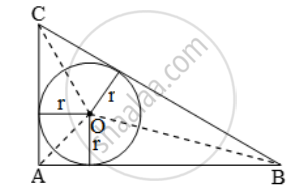# ABC is a right-angled triangle, right-angled at A. A circle is inscribed in it. The lengths of the two sides containing the right angle are 5 cm and 12 cm. Find the radius of the circle - Mathematics

Sum

ABC is a right-angled triangle, right-angled at A. A circle is inscribed in it. The lengths of the two sides containing the right angle are 5 cm and 12 cm. Find the radius of the circle

#### Solution

Given that ∆ABC is right angled at A.

AC = 5 cm and AB = 12 cm

BC2 = AC2 + AB2 = 25 + 144 = 169

BC = 13 cm

Join OA, OB, OCLet the radius of the inscribed circle be r

Area of ∆ABC = Area of ∆OAB + Area of ∆OBC + Area of ∆OCA

⇒ 1/2 × AB × AC

=\frac{1}{2}(12\times r )\text{}+\frac{1}{2}(13\times r )\text{}+\frac{1}{2}(5\times r )

⇒ 12 × 5 = r × {12 + 13 + 5}

⇒ 60 = r × 30 ⇒ r = 2 cm

Concept: Right-angled Triangles and Pythagoras Property
Is there an error in this question or solution?